# Integral domain

This article defines a property of commutative unital rings; a property that can be evaluated for a commutative unital ring
View all properties of commutative unital rings
VIEW RELATED: Commutative unital ring property implications | Commutative unital ring property non-implications |Commutative unital ring metaproperty satisfactions | Commutative unital ring metaproperty dissatisfactions | Commutative unital ring property satisfactions | Commutative unital ring property dissatisfactions
The property of being an ideal for which the quotient ring has this property is: prime ideal

## Definition

### Symbol-free definition

A commutative unital ring is termed an integral domain (sometimes just domain) if it satisfies the following equivalent conditions:

• It is a nonzero ring, and is cancellative
• The zero ideal is a prime ideal
• It is a nonzero ring, and the product of nonzero elements in nonzero
• It is a nonzero ring, and the product of nonzero ideals is nonzero
• It is a nonzero ring, and the set of nonzero elements is a saturated subset

### Definition with symbols

A commutative unital ring$R$ is termed an integral domain if$R$ satisfies the following equivalent conditions:

• Whenever$ab = ac$ and$a$ is not zero,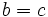$b = c$
• The ideal$0$ is a prime ideal
• Whenever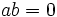$ab = 0$, either$a = 0$ or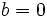$b = 0$

## Metaproperties

### Closure under taking the polynomial ring

This property of commutative unital rings is polynomial-closed: it is closed under the operation of taking the polynomial ring. In other words, if$R$ is a commutative unital ring satisfying the property, so is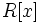$R[x]$

View other polynomial-closed properties of commutative unital rings

The polynomial ring over an integral domain is again an integral domain.

### Closure under taking subrings

Any subring of a commutative unital ring with this property, also has this property
View other subring-closed properties of commutative unital rings

Any subring of an integral domain is an integral domain. In fact, a commutative unital ring is an integral domain iff it occursas as a subring of a field.

### Closure under taking quotients

This property of commutative unital rings is not quotient-closed: in other words, a quotient of a commutative unital ring with this property, need not have this property

A quotient of an integral domain by an ideal need not be an integral domain; to see this, note that the quotient by an ideal is an integral domain iff the ideal is a prime ideal. Thus, the quotient of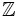$\mathbb{Z}$ by the non-prime ideal$6\mathbb{Z}$ is not an integral domain.

### Closure under taking localizations

This property of commutative unital rings is closed under taking localizations: the localization at a multiplicatively closed subset of a commutative unital ring with this property, also has this property. In particular, the localization at a prime ideal, and the localization at a maximal ideal, have the property.
View other localization-closed properties of commutative unital rings

The localization of an integral domain at any prime ideal is still an integral domain. More generally, the localization at any multiplicatively closed subset not containing zero, continues to be an integral domain. However, the converse is not true. If a commutative unital ring has the property that its localizations at all primes are integral domains, we can only conclude that it is a direct product of integral domains, and cannot deduce that it is itself an integral domain.

## Effect of property operators

### The quotient-closure

Applying the quotient-closure to this property gives: commutative unital ring

Any commutative unital ring can be expressed as a quotient of an integral domain by an ideal. In fact, any commutative unital ring can be expressed as a quotient of a polynomial ring over integers (a free commutative unital ring) by a suitable ideal.